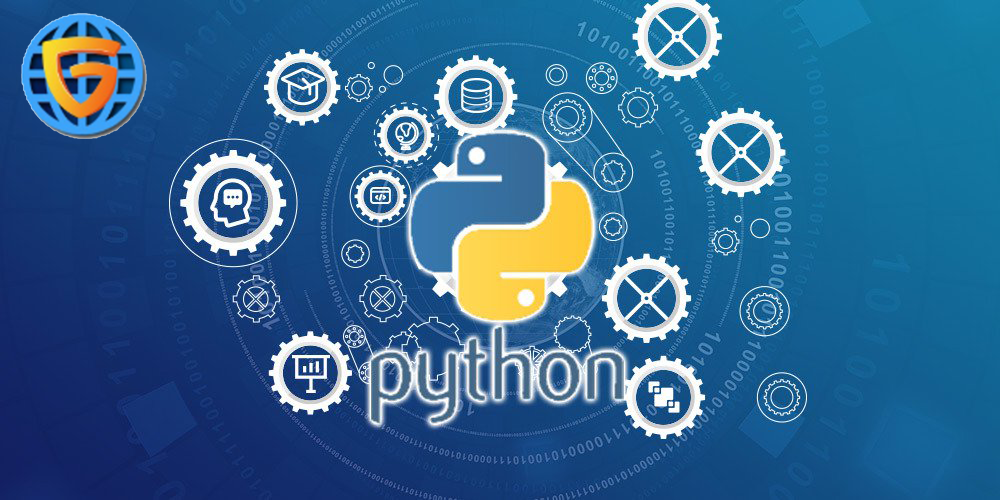## MACHINE LEARNING USING PYTHON

### MACHINE LEARNING USING PYTHON

• 30 July 2019Machine learning is the scientific study of algorithms and statistical models that computer systems use in order to perform a specific task effectively without using explicit instructions, relying on patterns and inference instead. It is seen as a subset of artificial intelligence

So the question comes that why are we using python in machine learning. The answer is simple. Python is a popular platform used for research and development of production systems. It is a vast language with number of modules, packages and libraries that provides multiple ways of achieving a task.

Python and its libraries like NumPy, SciPy, Scikit-Learn, Matplotlib are used in data science and data analysis. They are also extensively used for creating scalable machine learning algorithms. Python implements popular machine learning techniques such as Classification, Regression, Recommendation, and Clustering.

Python offers ready-made framework for performing data mining tasks on large volumes of data effectively in lesser time. It includes several implementations achieved through algorithms such as linear regression, logistic regression, Naïve Bayes, k-means, K nearest neighbor, and Random Forest.

###### Applications of Machine Learning Algorithms
1. Vision processing
2. Language processing
3. Forecasting things like stock market trends, weather
4. Pattern recognition
5. Games
6. Data mining
7. Expert systems
8. Robotics

So we are using python in machine learning because python have many libraries and packages which helps us to do that and make things very easy and simple. They can be imported very easily in python programming .Some of the packages which are needed in machine learning are:
• numpy - is used for its N-dimensional array objects
• pandas – is a data analysis library that includes dataframes
• matplotlib – is 2D plotting library for creating graphs and plots
• scikit-learn - the algorithms used for data analysis and data mining tasks
• seaborn – a data visualization library based on matplotlib

###### Concepts of Learning
Learning is the process of converting experience into expertise or knowledge.
Learning can be broadly classified into three categories, as mentioned below, based on the nature of the learning data and interaction between the learner and the environment.
• Supervised Learning
• Unsupervised Learning
• Semi-supervised learning

###### Similarly, there are four categories of machine learning algorithms as shown below:
• Supervised learning algorithm
• Unsupervised learning algorithm
• Semi-supervised learning algorithm
• Reinforcement learning algorithm
However, the most commonly used ones are supervised and unsupervised learning.